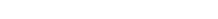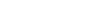AP Calculus Exam Scoring Guidelines

As you may already know, the AP Calculus exam consists of two main sections: multiple choice (MC) and free response (FR). In this article, you’ll learn about the AP Calculus exam scoring. Helpful hints included as a bonus!

AP Calculus Exam Scoring: Multiple Choice

The Multiple Choice section of the AP exam is similar in spirit to an SAT or ACT.

Following each question is a series of four potential answers. Fortunately, “none of the above” does not appear on this test, so you can be sure that one of the choices must be correct!

Scoring the MC Questions

Scoring the MC questions is straightforward. If you get the question right, you get 1 point. A wrong answer yields 0 points.

There is no penalty (negative points) for guessing. So try process of elimination if all else fails and maybe you can get it down to a choice between two or three answers.

In the worst case scenario, if you have no idea what a question is asking, take your chances on a random selection.Section II: Free Response

There are only six FR questions total in this section. So you can expect these problems to be time-consuming. In fact, the average amount of time per question in Section II should be about 15 minutes.

Each question typically has three or four related parts. Sometimes the various parts of the question rely on previous answers, but not always.

In fact, if you have no idea how to do the first part, write down something reasonable anyway and move on to the next parts. Sometimes points can be awarded for doing correct work on an incorrect function.

Scoring the FR Questions

Each FR question involves multiple parts and often many steps within each part as you work towards a final answer. There are 9 points possible for each question.

However, you must show your work! You can only earn points by showing the specific steps in a logical order that allow you to arrive at your final answers.

Even if you used a calculator function, such as numerical integration, you must at least write down the equation or formula on your test, as well as an indication of which calculator feature you used.

Example Rubric

Consider the following question taken from an AP Calculus BC test. We’ll see what goes into a perfect 9-point response.

For t ≥ 0, a particle is moving along a curve with its position at time t given by (x(t), y(t)) and dx/dt = 3t2 – 4, dy/dt = 2t. At time t = 1, its position is (-3, 1).

(a) Find the slope of the particle’s path at time t = 3.

(b) Find the x-coordinate of the particle’s position at time t = 5.

(c) Find the speed of the particle at time t = 5.

(d) Find the acceleration vector of the particle at time t = 5.

Solution

(a) Use the parametric slope formula, dy/dx = (dy/dt) / (dx/dt) = (2t) / ( 3t2 – 4 ). (1 point)

Plug in t = 3 to compute slope. (2(3)) / (3(3)22 – 4) = 6/23. (1 point)

(b) Integrate dx/dt = 3t2 – 4 with initial value x(1) = -3 to obtain x(t) = t3 – 4t. (2 points)

Plug in t = 5 to find x(5) = 105. (1 point)

(c) Determine velocity by plugging in t = 5 into the derivatives: v(5) = (71, 10). (1 point)

Speed is the magnitude of velocity:(1 point)

(d) Find second derivatives of x and y by differentiating the given derivatives.(1 point)

Plug in t = 5 to obtain (30, 2). (1 point)

AP Calculus Exam Scoring

Once all the scores are in, the points are tallied and converted into an overall score from 1 to 5.

Here is a short summary of the scoring process. More information can be found here: What is a Good AP Calculus Exam Score?

1. Section I (MC). 45 questions. Score one point for each correct response. Zero points for each incorrect. Raw score is then scaled up to 54 possible points.
2. Section II (FC). 6 multi-part questions. Score up to 9 points for each question depending on a detailed grading rubric. Up to 54 points available.

Improve your SAT or ACT score, guaranteed. Start your 1 Week Free Trial of Magoosh SAT Prep or your 1 Week Free Trial of Magoosh ACT Prep today!Author

•Shaun earned his Ph. D. in mathematics from The Ohio State University in 2008 (Go Bucks!!). He received his BA in Mathematics with a minor in computer science from Oberlin College in 2002. In addition, Shaun earned a B. Mus. from the Oberlin Conservatory in the same year, with a major in music composition. Shaun still loves music -- almost as much as math! -- and he (thinks he) can play piano, guitar, and bass. Shaun has taught and tutored students in mathematics for about a decade, and hopes his experience can help you to succeed!